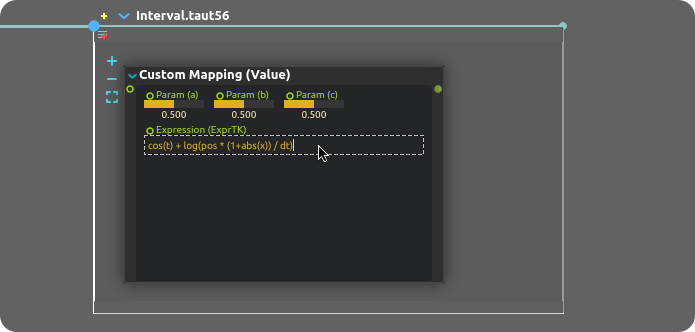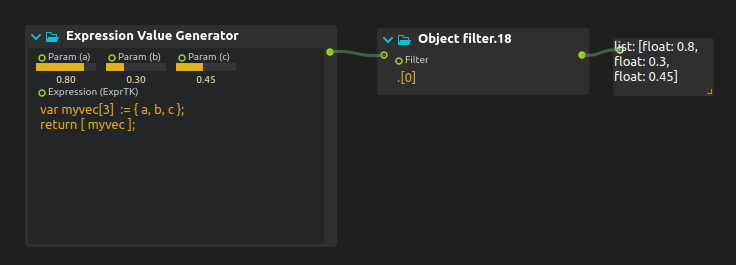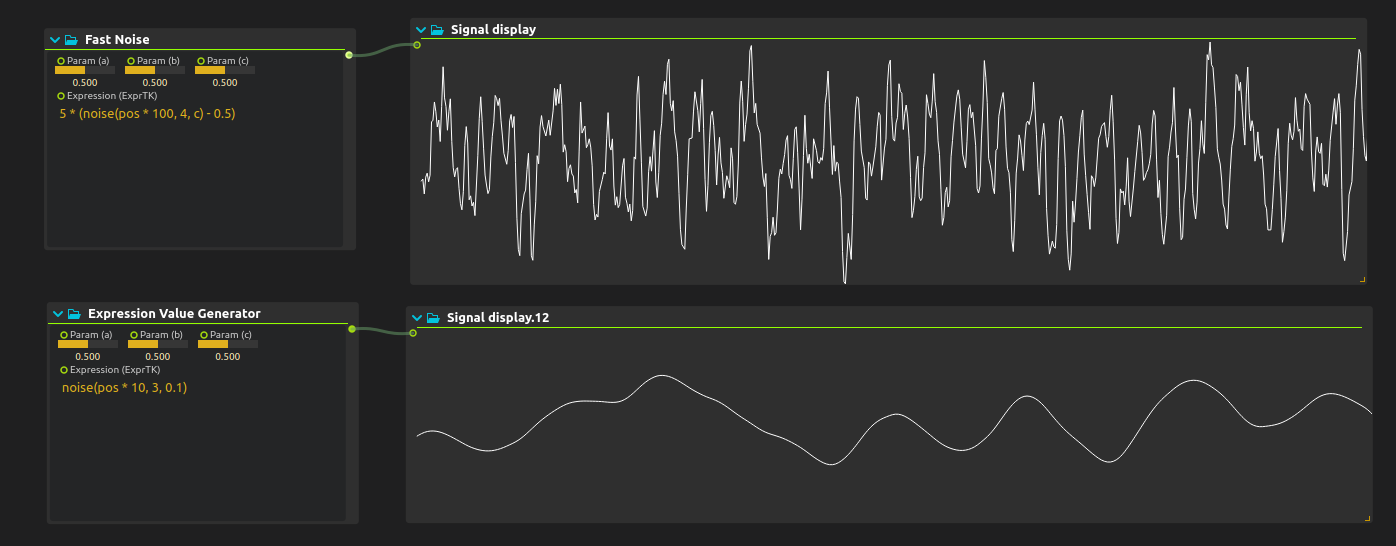# ExprTK support

Several processes allow to write simple math expressions. Such processes include:

• Micromap: for very simple math expressions, e.g. `x * 100`.
• Expression Audio Generator: generates multi-channel audio.
• Expression Audio Filter: filters multi-channel audio.
• Expression Value Generator: generates values suitable for the device explorer.
• Expression Value Filter: filters values suitable for the device explorer.These expressions are evaluated within score using the ExprTK library.

The expression can be written in the process built-in editor on multiple lines.

Numerous math functions are available: `sin`, `cos`, `abs`, `log`, as well as the usual `pi`, etc. constants.

## Audio handling

For audio processes, the processing is done per-frame. The input `x` is an array, where `x` is the first channel, `x` the second channel, etc.

Note that ExprTK has powerful array processing features; the following two codes implement the same distortion:

``````// This is the syntax to get the size of the array
// e.g. the number of channels here:
var n := x[];

for (var i := 0; i < n; i += 1) {
var dist := tan(x[i]*log(1 + 200 * a));
out[i] := clamp(-1, dist, 1);
}
``````
``````out := clamp(-1, tan(x * log(1 + 200 * a)), 1);
``````

## Array handling

For value processes, the same expression syntax cannot be used for single-value or array input:

• Use `x`, `px`, `po` to write an expression that processes single values, e.g. a float input.
• Use `xv`, `pxv`, `pov` to write an expression that processes arrays.

For instance:

``````tanh(x + 1)
``````

will process a single float as input, while:

``````return [ xv cos(xv), xv sin(xv) ];
``````

will take a vec2 that contains polar coordinates `[r, theta]` and convert it into cartesian coordinates `[x, y]`.

Note that it isn’t possible to create dynamic arrays in ExprTK. To return an array, it has to be wrapped in an array itself and then unwrapped with an Object filter:# Available variables

### Everywhere

• `t`: the current time
• `pos`: the position in the interval
• `a, b, c`: three provided controls
• `pa, pb, pc`: previous value of a, b, c
• `m1, m2, m3`: three provided variables (which will keep their value across a tick)
• In the audio cases they are arrays.

### Value mapping

• `x`: the value of the current input if it’s a single value
• `px`: the value of the previous input if it was a single value
• `po`: the value of the previous output if it was a single value
• `xv`: the value of the current input if it’s an array
• `pxv`: the value of the previous input if it was an array
• `pov`: the value of the previous output if it was an array
• `dt`: the time delta

### Audio mapping

• `x`: the value of the current sample
• `out`: where to write the output
• `px`: the value of the previous sample
• `fs`: the sampling rate

### Value generator

• `dt`: the time delta

### Audio generator

• `out`: where to write the output
• `fs`: the sampling rate

# Available functions

## ExprTK-provided functions:

• Basic math: `min, max, avg, sum, abs, ceil, floor, round, roundn, exp, log, log10, logn, pow, root, sqrt, clamp, inrange, swap`
• Trigonometry: `sin, cos, tan, acos, asin, atan, atan2, cosh, cot, csc, sec, sinh, tanh, d2r, r2d, d2g, g2d, hyp`
• Numeric integration and differentiation
• Vector Processing: `BLAS-L1 (axpy, axpby, axpb), all/any-true/false, count, rotate-left/right, shift-left/right, sort, nth_element, iota, sum, kahan-sum, dot-product, copy`

## score-provided functions

• `random(min, max)`: returns a random real number in the given interval.
• `random(0, 1)` can be 0., 0.3, 0.23455436, 1.
• `round(random(1, 20))` is a 20-sided dice with lower probabilities for 1, 20.
• `floor(random(1, 21))` is a 20-sided dice with same probabilities for everyone.
• `noise(t, octaves, persistence)`: Perlin noise.
• `t` should be something that increases ; `pos` is generally a good candidate.
• `octaves`: the higher this is, the more detailed the noise is. 2 would be smooth, 7 very detailed but more computationally intensive. Between 1 and 10.
• `persistence`: how much each octave fades. 1: no fade, very noisy. 0: very smooth.
• `5 * (noise(pos * 100, 4, 0.5) - 0.5)` gives a convincing fairly dynamic noise between -1 and 1.
• `noise(pos * 10, 3, 0.1)` gives a very smooth evolution between 0 and 1.# Examples

The user library comes with a few utility functions, be sure to check them in the preset pane !

Contributing useful functions to the library is also very welcome.

## Value generator

### Logistic function

Implemented as a preset (`Logistic`):

``````if(m1 == 0) {
m2 := 0.8;
m1 := 1;
}

var r := 4 * a;
m2 := r * m2 * (1 - m2)
``````

## Value mapper

Implemented as a preset (`Noisify`):

``````var rnd_m := pow(2, 31);
var rnd_a := 1103515245;
var rnd_c := 12345;

if(m2 == 0) {
m2 := 1;
m1 := 12345678;
}

var r := (rnd_a * m1 + rnd_c) % rnd_m;
m1 := r;
x + a * r / (2^33);
``````

## Audio generator

### Sine wave

Implemented as a preset (`Sine`):

``````var phi := 2 * pi * (20 + a * 500) / fs;

m1 += phi;

out := b * cos(m1);
out := b * cos(m1);
``````

### Square wave

Implemented as a preset (`Square`):

``````var phi := 2 * pi * (20 + a * 500) / fs;

m1 += phi;

var f := cos(m1) > 0 ? b : -b;
out := f;
out := f;
``````

### Wobbly synth

Implemented as a preset (`Wobbly`):

``````var freq_l := 225 +  cos(t/(100*(a+0.1)));
freq_l := 2 * pi * m1 *  freq_l * b / fs;

var freq_r := 215 +  sin(t/(100*(a+0.1)));
freq_r := 2 * pi * m1 *  freq_l * b / fs;

m1 += 1;

out := b * cos( freq_l );
out := b * cos( freq_r );
``````

## Audio filter

### Crude distortion

2-channel version:

``````out := clamp(0,  tan(x*log(1 + 200 * a)), 1);
out := clamp(0,  tan(x*log(1 + 200 * a)), 1);
``````

Any number of channel version:

``````var n := x[];

for (var i := 0; i < n; i += 1) {
var dist := tan(x[i]*log(1 + 200 * a));
out[i] := clamp(-1, dist, 1);
}
``````